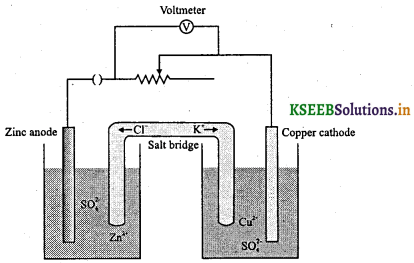# 1st PUC Chemistry Question Bank Chapter 8 Redox Reactions

Students can Download Chemistry Chapter 8 Redox Reactions Questions and Answers, Notes Pdf, 1st PUC Chemistry Question Bank with Answers helps you to revise the complete Karnataka State Board Syllabus and to clear all their doubts, score well in final exams

## Karnataka 1st PUC Chemistry Question Bank Chapter 8 Redox Reactions

Question 1.
What are redox reactions?
The reactions in which both oxidation and reduction reactions take place are called redox reactions.

Classical idea of redox reactions-Oxidation and reduction reactions

Question 1.
What is oxidation? Give examples.
Oxidation is defined as the addition of oxygen / electronegative element to a substance or removal of hydrogen / electropositive element from a substance.
2Mg + O2 → 2MgO (oxidation of magnesium)

b) Removal of hydrogen:
H2S + Cl2 → 2HCl + S (oxidation of H2S)

2Mg + Cl2 → MgCl2 (oxidation of magnesium)

d) Removal of electropositive element:
2K4[Fe(CN)6(aq) + H2O2(aq) → 2K3[Fe(CN)6](aq) + 2KOH(aq) (Removal of electropositive potassium)Question 2.
What is reduction? Give examples.
Reduction is defined as the removal of oxygen/electronegative element from a substance or addition of hydrogen/electropositive element to a substance. Examples:
a) Removal of oxygen:
2HgO(s) → 2Hg(l) + O2(g) (Reduction of mercuric oxide)

b) Removal of electronegative element:
2FeCl3 + H2 → 2FeCl2 + 2HCl (Reduction of ferric chloride)

CH2 = CH2 + H2 → CH3 – CH3 (Reduction of ethene)

2HgCl2 + SnCl2 → Hg2Cl2 + SnCl4 (Reduction of mercuric chloride)

Redox Reactions in Terms of Electron Transfer Reactions

Question 1.
Define oxidation interms of electron transfer. Give an example.
The reaction that involves loss of electrons is called oxidation.
Example: Na → Na+ + e

Question 2.
Define reduction in terms of electron transfer. Give an example.
The reaction that involves gain of electrons is called reduction.
Example: Cl2 + 2e → 2Cl

Question 3.
Define redox reaction in terms of electron transfer. Give an example.
A reaction during which loss of electrons by one substance and gain of electrons by other substance takes place simultaneously is called redox reaction.Question 4.
Define reducing agent and oxidizing agent with an illustration.
A substance that can readily lose electrons to other substance is called reducing agent or reductant.
A substance that can readily accept electrons from other substance is called oxidising agent or oxidant. So, reducing agents are electron donors and oxidizing agents are electron acceptors.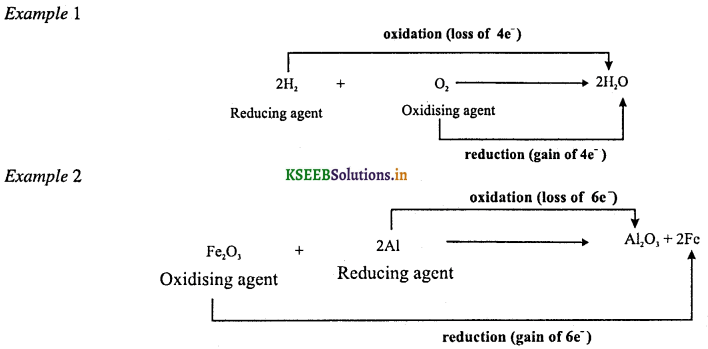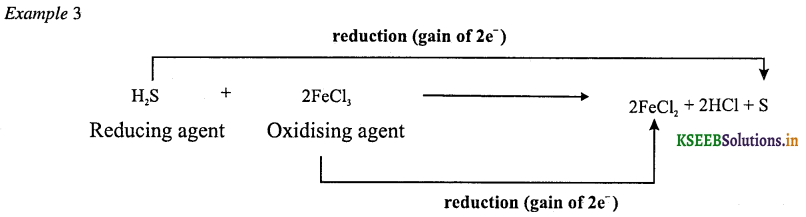Question 5.
What is electrochemical series?
A table representing various metal or ions which are arranged in the decreasing order of their tendency to release electrons (or increasing order of their SRP values) is called electrochemical series.
Example: Tendency of releasing electrons in Cu, Zn and Ag in the order: Zn > Cu > Ag.

Oxidation Number

Question 1.
What is oxidation number?
It is an apparent or real charge on one atom of the element-

Question 2.
Rule for assigning oxidation numbers.
1. The oxidation number of an atom in the elemental state (uncombined state) is zero.
Examples: Oxidation number of each atom in Cl2, H2, O3, S8 Na, Mg, Al is zero.

2. The oxidation number of an ion is equal to its charge.
Examples: Na+ has an oxidation number of +1, Cl has -1, Mg2+ has +2.

3. The oxidation number of hydrogen in all compounds containing hydrogen is +1, except when hydrogen forms compounds called hydrides with active metals in which it is -1.
Example: Oxidation state of H is +1 in H2O, but -1 in NaH (sodium hydride).

4. The oxidation number of oxygen in all compounds containing oxygen is -2, except in peroxides in which it is -1, and super oxides in which it is $$-\frac { 1 }{ 2 }$$ .
Example: In H2O, the oxidation state of oxygen is -2, and in H2O2 it is -1.
The oxidation state of oxygen in OF2 and O2F2 are + 2 and + 1 respectively.

5. The algebraic sum of the oxidation numbers of all the atoms present in one molecule of a compound is zero.

6. The algebraic sum of the oxidation numbers of all the atoms in the formula for a polyatomic ion is equal to the charge on that ion.

7. The oxidation state of halogen in their compounds is – 1 but in interhalogen compounds of Cl, Br and I, the more electronegative of the two halogens gets the oxidation number of -1.
For example, in BrCl3, the oxidation number of Cl is -1 while that of Br is +3.
When Cl, Br and I combined with oxygen atom, for example, in oxoacids and oxoanions, halogens have positive oxidation numbers.

8. The oxidation number of alkali metals in their compounds is +1, and that of alkaline earth metals in their compounds is +2.
Example: Oxidation state of K in KMnO4 is +1.Question 3.
Calculate the oxidation number of underlined atom in the following compound.
a) H2SO4
Let ‘x’ be the oxidation number of sulphur atom
2 (+1) + x + 4 (-2) = 0
2 + x – 8 = 0
x = +6

b) HNO3
Let ‘x’ be the oxidation number of nitrogen atom
1 (+ 1) + x + 3(-2) = 0
1 + x – 6 = 0
x = +5

c) K2Cr2O7
Let ‘x’ be the oxidation number of chromium atom
2(+1) + 2x + 7(-2) =0
2 + 2x – 14 = 0
2x = 12 ⇒ x = +6

d) KMnO4
Let ‘x’ be the oxidation number of manganese atom
1(+1) + x + 4 (-2) = 0
1 + x – 8 = 0
x = +7

e) Fe2O3
Let ‘x’ be the oxidation number of iron atom
2x + 3 (-2) = 0
2x – 6 = 0
2x = 6 ⇒ x = +3

f) Mn2O7
Let ‘x’ be the oxidation number of manganese atom
2x + 7(- 2) = 0
2x – 14 = 0
2x = 14 ⇒ x = +7

g) H2S2O7
Let ‘x’ be the oxidation number of sulphur atom
2(+1) + 2x + 7(-2) = 0
2 + 2x – 14 = 0
2x = 12 ⇒ x = + 6Question 4.
Calculate the oxidation number of underlined atom in the following ionic species.
a) MnO4
x + 4(-2) = -1
x – 8 = -1
x = +7

b) Cr2O72-
2x + 7 (-2) = -2
2x -14 = -2
2x = 12 ⇒ x = +6

c) HSO4
1 (+1) + x + 4 (- 2) = -1
1 + x – 8 = -1
1 + x = + 7
x = + 6

d) CO32-
x + 3 (-2) = -2
X – 6 = -2
x = +4

Question 5.
Consider the elements: Cs, Ne, I and F
(a) Identify the element that exhibits only negative oxidation state.
(b) Identify the element that exhibits only positive oxidation state.
(c) Identify the element that exhibits both positive and negative oxidation states.
(d) Identify the element which exhibits neither the negative nor the positive oxidation state.
(a) F (Fluorine) exhibits only -ve oxidation state (-1) in its compounds because it is the most electronegative element.

(b) Cs (Caesium) exhibits only + ve oxidation state (+1) in its compounds because it is the most electropositive element.

(c) Iodine has seven electrons in the valence shell as well as vacant 5d orbital to which electrons from 5p and 5s orbitals can be shifted. Therefore, this element exhibits – 1 oxidation state as well as variable positive oxidation states of +1,+3, and +5, +7 in its compounds.

(d) Ne (neon) neither exhibits + ve nor -ve oxidation states because it is a noble gas element with completely filled orbitals (1s2, 2s2, 2p6). Moreover, it has no vacant d – orbitals.Question 6.
What is stock notation? Give examples.
Representation of oxidation number by putting a Roman numeral in parenthesis after the symbol of the metal in molecular formula is called stock notations.

Question 7.
Using stock notation represent the following compounds: HAUCl4, Ti2O, FeO, Fe2O3, CuI. CuO, MnO and MnO2. (NCERT solved problem)
By applying various rules of calculating the oxidation number of the desired elements in a compound, the oxidation number of each mi tallic element in its compound is as follows:
(i) HAuCl4 = 0
1 + x + 4 (-1) = 0
x = + 3

(ii) Ti2O = 0
2(x) + (-2) = 0
2x = + 2
x = + 1

(iii) FeO = 0
x + (-2) = 0
x = 2

(iv) Fe2O3 = 0
2(x) + 3 (-2) = 0
2x – 6 = 3
x = + 3

(v) CuI = 0
x + (-1) = 0
x = + 1

(vi) CuO = 0
x + (-2) = 0
x = + 2

(vii) MnO = 0
x + (-2) = 0
x = + 2

(viii) MnO2 = 0
x + 2 (-2) = 0
x = +4
Therefore, these compounds may be represented as:
HAu(III)Cl4, Tl2(I)O, Fe(II)0, Fe2(III)O3, Cu(I)I, Cu(II)O, Mn(II)O, Mn(IV)O2.Question 8.
Write formulas for the following compounds:
(a) Mercury (II) chloride
(b) Nickel (II) Sulphate
(c) Tin (IV) oxide
(d) Thallium (I) Sulphate
(e) Iron (III) sulphate
(f) Chromium (III) oxide
(a) HgCl2
(b) NiSO4
(c) SnO2
(d) Tl2SO4
(e) Fe2(SO4)3
(f) Cr2O3

Question 9.
Define oxidation interms of oxidation number.
An increase in the oxidation number of the element in the given substance is called oxidation.

Question 10.
Define reduction interms of oxidation number.
A decrease in the oxidation number of the element in the given substance is called reduction.

Question 11.
Define reducing agent and oxidizing agent interms of oxidation number with an illustration.
A reagent which decreases the oxidation number of an element in another reactant is called reducing agent. The oxidation number of reducing agent is increased during redox reaction.
A reagent which increases the oxidation number of an element in another reactant is called oxidising agent. The oxidation number of oxidizing agent is decreased during redox reaction.
For example,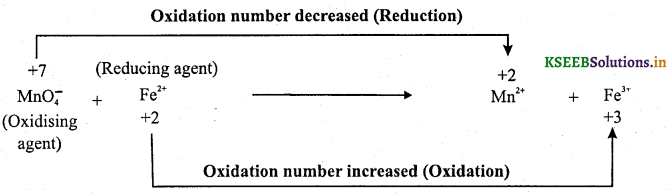Question 12.
Justify that the reaction: 2Cu2O(s) + Cu2S(s) → 6Cu(s) + SO2(g) is a redox reaction. Identify the species oxidised/reduced, which acts as an oxidant and which acts as a reductant.In this reaction copper is reduced from +1 state to zero oxidation state and sulphur is oxidised from -2 state to +4 state. Hence, Cu(I) is an oxidant and sulphur of Cu2S is reductant.

Question 13.
What is Combination reaction? Illustrate with an example.
Redox reaction in which chemical compounds are formed by combination of two or more elements is called combination reaction.
Examples:Question 14.
What are decomposition reactions? Illustrate with an example.
These are redox reactions in which chemical compounds undergo decomposition (by itself/heating) to give products containing at least one elemental state.
Examples,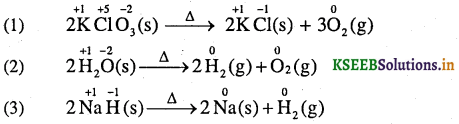Question 15.
What are displacement redox reactions? Illustrate with an example.
These are redox reaction in which an atom/ion present in the chemical compound gets replaced by atom/ion of another element.
X + YZ → XY + Z

Question 16.
Give an example for metal displacement reaction.
A metal in a compound can be displaced by another metal in the uncombined state.
Examples:Question 17.
Give example for non-metal displacement reaction.
All alkali metals and some alkaline earth metals which are very good reductants will displace hydrogen from cold water.
ExamplesFluorine is so reactive that it attacks water and displaces the oxygen of water.
ExampleQuestion 18.
What is disproportionation reaction? Give an example.
It is a specific type of redox reaction in which a species is simultaneously reduced and oxidized to form two different products.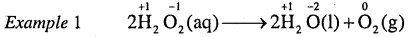Here the oxygen of hydrogen peroxide, which is present in -1 state, is converted to zero oxidation state in O2 and decreases to -2 oxidation states in H2O.Question 19*.
In the reactions given below, identify the species undergoing oxidation and reduction:
(i) H2S(g) + Cl2(g) → 2HCl(g) + S(s)
(ii) 3Fe3O4(s) + 8Al(s) → 9Fe(s) + 4Al2O3(s)
(iii) 2Na(s) + H2(g) → 2NaH(s)
(i) H2S is oxidised because a more electronegative element, chlorine is added to hydrogen (or a more electropositive element, hydrogen has been removed from S). Chlorine is reduced due to addition of hydrogen to it.

(ii) Aluminium is oxidised because oxygen is added to it. Ferrous ferric oxide (Fe3O4) is reduced because oxygen has been removed from it.

(iii) Sodium is oxidised and hydrogen is reduced.

Question 20.
Justify that the reaction 2Na(s) + H2(g) → 2NaH(s) is a redox change.Splitting of the reaction under examination into two half reactions reveals that here sodium is oxidised and hydrogen is reduced, therefore, the complete reaction is a redox change.

Question 21.
Which of the following species do not show disproportionation reaction and why?
ClO, ClO2, ClO3 and ClO4 Also write reaction for each of the species that disproportionates.
Among the oxoanions of chlorine given above, ClO4 does not disproportionate because in this oxoanion chlorine is present in its highest oxidation state that is, +7. The disproportionation reactions for the other three oxoanions of chlorine are as follows:Question 22.
Suggest a scheme of classification of the following redox reactions.
(a) N2(g) + O2(g) → 2NO(g)
(b) 2Pb(NO3)2(g) → 2PbO(s) + 2NO2(g) + $$\frac { 1 }{ 2 }$$ O2(g)
(c) NaH(s) + H2O(1) → NaOH(aq) + H2 (g)
(d) 2NO2(g) + 2OH(aq) → NO2(aq) + NO3(aq) + H2O(I)
In reaction (a), the compound nitric oxide is formed by the combination of the elemental substances, nitrogen and oxygen; therefore, this is an example of combination redox reactions.
The reaction (b) involves the breaking down of lead nitrate into three components; therefore, this is categorised under decomposition redox reaction.
In reaction (c), hydrogen of water has been displaced by hydride ion into dihydrogen gas. Therefore, this may be called as displacement redox reaction. The reaction (d) involves disproportionation of NO2 (+4 state) into NO2 (+3 state) and NO3 (+ 5 state). Therefore reaction (d) is an example of disproportionation redox reaction.Question 23.
Give the different steps involved in the balancing of redox equations by oxidation number method.
Balancing redox reactions by oxidation number method was developed by Johnson.
Steps involved in the balancing of redox equations by oxidation number method.
Step 1: Write the skeletal equation (if not given frame it) of all the reactants and products of the reaction.

Step 2: Indicate the oxidation number of each element in the equation and identify the elements which undergo a change in the oxidation number.

Step 3: Calculate the increase or decrease in oxidation number per atom and identify the oxidising and reducing agents.

Step 4: Multiply the formulae of the oxidising and the reducing agents by suitable integers so as to equalise the total increase or decrease in oxidation number.

Step 5: Balance the number of atoms other than oxygen and hydrogen atoms.

Step 6: Balance hydrogen and oxygen atoms by H2O molecules using hit and trial method.

(a) In acidic and neutral solutions: First balance O atoms by adding H2O molecules to whatever side deficient in O atoms and then balance hydrogen atoms by adding H+ ions to whatever side deficient in hydrogen atoms.

(b) For basic medium: First balance oxygen atoms by adding H2O molecules to whatever side deficient in oxygen atoms. The hydrogen atoms are then balanced by adding H2Omolecules equal in number to the defficiency of hydrogen atoms and an equal number of OH ions are added to the opposite side of the equation.

Question 24.
Balance the following redox reaction by oxidation number method
Cr2O2-7 + SO2-3 → Cr3+ + SO2-4 + H2O (Acid medium)
Step 1: Skeleton equation is
Cr2O72- + SO32- → Cr3+ + SO42- + H2O

Step 2: Assign the oxidation number and find the change in oxidation number for the atoms undergoing oxidation and reduction.Step 3: Equalise the change in oxidation number for both the atoms by multiplying with suitable integers and the number of atoms other than oxygen and hydrogen.
Cr2O72- + 3SO32- → 2Cr3+ + 3SO42- + H2O

Step 4: Add H+ and water to balance oxygen and hydrogen atoms in acidic medium.
Cr2O72- + 3SO32- + 8H+ → 2Cr3+ + 3SO42- + 4H2OQuestion 25.
Balance the following redox reaction by oxidation number method.
MnO2 + Br → Mn2+ + Br2 + H2O (Acid medium)
Step 1: Skeleton equation is
MnO2 + Br → Mn2+ + Br2 + H2O

Step 2: Assign the oxidation number and find the change in oxidation number for the atoms undergoing oxidation and reduction.Step 3: Equalise the change in oxidation number for both the atoms by multiplying with suitable integers and balance the number of atoms other than oxygen and hydrogen.
MnO2 + 2Br → Mn2+ + Br2 + H2O

Step 4: Add H+ and water to balance oxygen and hydrogen atoms in acidic medium.
MnO2 + 2Br + 4H+ → Mn2+ + Br2 + H2O

Question 26.
Give the different steps involved in the balancing of redox equations by half reaction method (ion – electron method).
In this method, the reaction is broken down into two half-reactions, one for oxidation and another for reduction. It involves the following steps:
1. For the given skeleton equation (if not given, frame it), assign oxidation numbers to the atoms in the equation and find out which atoms are undergoing oxidation and reduction.

2. Split the redox reaction into two half-reactions, one for oxidation and other for reduction and balance the atoms other than H and O for each half-reaction using simple multiples. Remove the electrons from the oxidation half-reaction (equal to increase in oxidation number per atom) and add the electrons to the reduction half reaction (equal to decrease in oxidation number per atom).

3. Multiply oxidation equation by added electrons of reduction half-reaction and reduction equation by removed electrons of oxidation half-reaction so that number of electrons becomes equal in both the half-equations. Then add.

4. Balance hydrogen and oxygen atoms by H2O molecules using hit and trial method.
(a) In acidic and neutral solutions: First balance O atoms by adding H2O molecules to whatever side deficient in O atoms and then balance hydrogen atoms by adding H+ ions to whatever side deficient in hydrogen atoms.

(b) For basic medium: First balance oxygen atoms by adding H2O molecules to whatever side deficient in oxygen atoms. The hydrogen atoms are then balanced by adding H2O molecules equal in number to the defficiency of hydrogen atoms and an equal number of OH ions are added to the opposite side of the equation.Question 27.
Balance the following redox reaction by half reaction method
Fe2+(aq) + Cr2O72- (aq) → Fe3+ (aq) + Cr3+(aq) (Acid medium)
Step 1: For the given skeleton equation (if not given, frame it), assign oxidation numbers to the atoms in the equation and find out which atoms are undergoing oxidation and reduction.Step 2: Separate the equation into half reactions: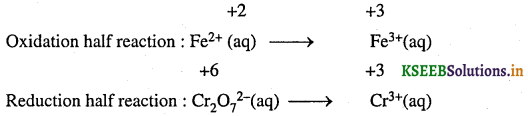Step 3: Balance the atoms other than O and H in each half reaction individually. Here the oxidation half reaction is already balanced with respect to Fe atoms. For the reduction half reaction, we multiply the Cr3+ by 2 to balance Cr atoms.
Fe2+ (aq) → Fe3+ (aq)
Cr2O72-(aq) → 2Cr3+(aq)

Step 4: For reactions occurring in acidic medium, add H2O to balance O atoms and H+ to balance H atoms. Thus, we get:
Fe2+(aq) + Cr2O72- (aq) + 14 H+ → Fe3+ (aq) + 3Cr3+(aq) + 7H2O

Step 5: Add electrons in respective side of the half reactions to balance the charges. Thus oxidation and reduction half reactions are written to balance the charge as:
Fe2+ (aq) → Fe3+(aq) + e
Cr2O72-(aq) + 14H+ (aq) + 6e → 2Cr3+(aq) + 7H2O (1)

To equalise the number of electrons in both the half reactions, multiply the oxidation half reaction by 6 and write as:
6Fe2+ (aq) → 6Fe3+(aq) + 6e

Step 6: Add the two half reactions to get the overall reaction and cancel the electrons on each side. This gives the net ionic equation as :
6Fe2+(aq) + Cr2O72-(aq) + 14H+(aq) → 6 Fe3+(aq) + 2Cr3+(aq) + 7H2O(l)Question 28.
Permanganate(VII) ion, MnO4 MnO4– in basic solution oxidises iodide ion, I- to produce molecular iodine (I2) and manganese (IV) oxide (MnO2). Write a balanced ionic equation to represent this redox reaction.
or
Balance the following redox reaction by half reaction method MnO4 (aq) + I (aq) → MnO2(s) + I2(s)
(Basic medium)
Step 1: For the given skeleton equation (if not given, frame it), assign oxidation numbers to the atoms in the equation and find out which atoms are undergoing oxidation and reduction.Step 2: Separate the equation into half reactions:
Oxidation half reaction: I(aq) → I2 (s)
Reduction half reaction: MnO4(aq) → MnO2(s)

Step 3: Balance the atoms other than O and H in each half reaction individually. The number of Mn atoms is balanced in reduction half reaction. In oxidation half reaction, I- is multiplied by 2 to balance I atoms.
2I(aq) → I2(s)

Step 4: To balance the O atoms in the reduction half reaction, add two water molecules on the right:
MnO4 (aq) → MnO2 (s) + 2 H2O (l)
To Balance the ‘H’ atoms, add 4 H2O molecules to the left and 4OH to the right.
MnO4 (aq) + 4 H2O → MnO2(s) + 2H2O (l) + 4OH
Then cancel H2O molecules common in both sides.
MnO4 (aq) + 2H2O (1) → MnO2 (s) + 4 OH (aq)

Step 5: Add electrons in respective side of the half reactions to balance the charges. Thus oxidation and reduction half reactions are written to balance the charge as: 2I (aq) → I22 (s) + 2e
MnO4(aq) + 2H2O(1) + 3e → MnO2(s) + 4OH(aq)
To equalise the number of electrons in both the half reactions, we multiply the oxidation half reaction by 3 and reduction half reaction by 2 and write as : 6I (aq) → 3I2 (s) + 6e
2MnO4(aq) + 4H2O(1) + 6e → 2MnO2(s) + 8OH(aq)

Step 6: Add the two half reactions to get the overall reaction and cancel the electrons on each side. This gives the net ionic equation as :
2MnO4 (aq) + 6l (aq) + 4H2O → 2MnO2(s) + 3I2s) + 8OHQuestion 29.
What is electrode potential?
The potential developed when a metal is in equilibrium with a solution of its own ions is called electrode potential.

Question 30.
What is standard electrode potential?
The potential developed when a metal is in equilibrium with one molar solution of its own ions at 298K is called-standard electrode potential.

Question 31.
What is redox couple?